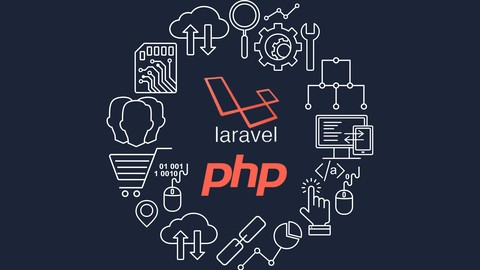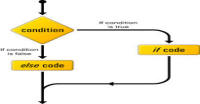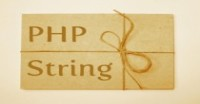# Array and foreach loop in PHP

In this tutorial, we will study about array and foreach loop. Let's assume you have to store large data, for example first 10000 multiples of five. Then you will have to define 10000 variables with 10000 names. It will make your task very tough. One of the solution is storing data in array. We can store many values just in single array. Let's take a look how?

```<?php
// defining an array with three values
\$type1 = array('first element', 'second element', 'third element');

// for printing full array we use  print_r function
// display => Array (  => first element  => second element  => third element )
print_r(\$type1);

echo "
";

// for printing single element we use indexes
// for \$type1 indexes are 0, 1, 2
echo \$type1;
echo "
";
echo \$type1;
echo "
";

echo \$type1;
echo "
";

?>
```
• In line 3, we are defining an array (named \$type1) and filling 3 values ('first element', 'second element', 'third element') in it.
• Now how will we use it? Every value is mapped to an unique identity, called index. We will access these values via indexes. Line 7 is printing the array using print_r() function. You can see that you have filled just values but you are getting that numbers starting from 0 are mapping to those values. In fact these are position number (in programming position starts from 0 instead of 1).
• In line 13, 15 and 18, we are printing the values which we have stored on the position 0, 1 and 2 respectively.

### Associative array:

In above example, we use the default indexes ( 0, 1 and 2). We can also define our own indexes just like in this example.

```<?php
// second type to define array
\$type2 = array('key'=>'value','key2'=>'value2','key3'=> 'value3');

// display => Array ( [key] => value [key2] => value2 [key3] => value3 )
print_r(\$type2);

echo "
";
//for \$type2 indexes are key, key2, keys3
echo \$type2['key3'];

?>
```

Here we are also defining own own indexes (keys) which are mapped with values. This types of arrays are called associative array.

### Accessing the arrays:

Every value in the array is an individual variable. We can operate all operations which we can do on variables ( like addition, subtraction, assigning values, etc.)

```<?php

// we are defining an empty array (no data)
\$arr = array();

// we can assign data like this
\$arr = 'harish';
\$arr = 'kumar';

// we can also skip indexes ( there is no 2 and 3)
\$arr = 'jump';

// we can use both types of indexes in same array
\$arr['day'] = 'Monday';
\$arr['num1'] = 4;
\$arr['num2'] = 5;

// each variable is independent
\$arr['num3'] = \$arr['num1'] + \$arr['num2'];

// printing array
print_r(\$arr);

?>
```

Note: We can define array inside an array itself. It is called multidimensional array. For example:

\$a = array(1, array(2, 3), 4);

### Array with loops:

Lets assume we have to do some operation on a large data stored in array (say printing data or adding numbers). For this work we will use loops. Now take an example of for loop:

```<?php

\$a = array(1,21,23,43,32,23,4);
// our variables are \$a, \$a, \$a........

// initially sum is 0
\$sum = 0;
for(\$i = 0;\$i<7;\$i++)
{
// i will increase 1 every time, i.e. position of array will shift
// 1 every time in loop
\$sum += \$a[\$i];
}
echo \$sum;

?>
```

This is your exercise to do this example using while and do while loop.

There is one more loop in PHP, specially for array, called foreach loop. Foreach loop is a loop which start some operation from first element (data) and does up to last element. Lets do above example with foreach loop.

```<?php

\$a = array(1,21,23,43,32,23,4);
// our variables are \$a, \$a, \$a........

// initially sum is 0
\$sum = 0;
foreach (\$a as \$v) {
// in each iteration we are doning something like this
// \$v = \$a[\$i];
// \$sum += \$v;
// i++;
\$sum += \$v;
}
echo \$sum;

?>
```

Here we are defining a new variable \$v each time and giving the value of next element of \$a. Foreach loop is useful when you don't know how many elements are in the array or when you are using associative array.

### Foreach loop with associative array:

In the case of associative array we have to define two variables (instead of one) because we don't know the index (key) for each element. First variable is the index and another is value mapped with it.

```<?php

\$a = array('name'=>'harish','soms' => 'word','somemore' => 'title','author' => 'harish','last' => 'kumar');

foreach (\$a as \$k => \$v) {
// observe the value of \$k and \$v
echo "key is \$k and value is \$v
";
}

?>
```

If you find any difficulty in this tutorial or you have some suggestion then please leave comment. In next tutorial we will study about the operations on the arrays.

Don't forget to Subscribe for latest feeds.

Show full series till now

Go Back To Last Tutorial

#### Click on banner below to Learn: PHP with Laravel for beginners - Become a Master in LaravelHarish, a technical core team member at www.lyflink.com with five year experience in full stack web and mobile development, spends most of his time on coding, reading, analysing and curiously following businesses environments. He is a non-graduate alumni from IIT Roorkee, Computer Science and frequently writes on both technical and business topics.

### Related ArticlesPHP stands for PHP: Hypertext Preprocessor. PHP is known as a server side scripting and interpreter language for web ...
##### Introduction to PHPIn this tutorial, we will study about conditional statements (if else statements and switch statements). These statem...
##### Conditional statements in phpIn this tutorial, we will study about strings and operations upon strings. Due to long length of this section, I have...

### The Complete Web Developer Course - Build 25 Websites